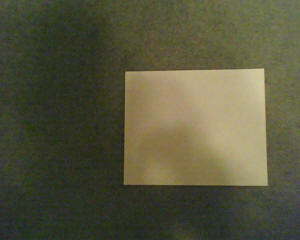How to measure the AOV (Angle of View) and focal length of the lens (35mm-film equivalent)
130703 - There are two ways to measure AOV.
1. Letter Paper - This method is easy to do but is accurate only for rectilinear lenses, not fisheye lenses.  A rectilinear lens will record straight horizontal and vertical lines as straight lines.  A fisheye lens will record some straight lines as curved lines.
2. Tape Measure - This method is accurate for both rectilinear and fisheye lenses.  To measure Horizontal Angle Of View (HAOV) take a landscape photo or video of a horizontal tape measure from a measured distance (X).  Make sure that the tape measure intersects the center of the image.  Y becomes the width of the tape measure in the image.  W and Z are equal, and are the image width in pixels.

130602 - This AOV spreadsheet XLS file will do the calculations for you, no calculator required.  Download it and open it with Excel or any XLS compatible spreadsheet program like Google Docs online spreadsheet.

The 16:9 diagonal, horizontal and vertical AOV ratios are 1.15, 1, 0.526
The 4:3  diagonal, horizontal and vertical AOV ratios are 1.2, 1, 0.75

This is a procedure to measure the horizontal AOV (angle of view) of an 808 camera.  You will take a video or photo of a standard piece of paper from a known distance.  You will need a scientific calculator.  The vertical angle of view and the diagonal angle of view are not measured here.

For reference, a 35mm camera has a 36x24mm film image size. With a 50mm lens the camera has a diagonal, horizontal and vertical AOV of 47, 40 and 27 degrees, respectively.

In 808 cameras, the AOV of a video frame and a photo frame can be different.  You need to decide which one, or both, you want to measure.
If a video frame, you need to take a short video of a paper sample, then use a software tool (like VirtualDub) to extract a frame to a JPG image, and then measure the pixels in the JPG image using a photo editor.
If a photo frame
, you will measure the pixels in a JPG image using a photo editor.

 1. Take a landscape photo of a landscape piece of letter or A4 paper from a (X) fixed distance (36 inches or 1000 millimeters).  (Y) will be the paper width (letter 11 inches or A4 297 mm). Center the paper best you can.   As long as measurements X and Y are in the same units (inches or millimeters) the calculations will work. 2. Use a graphics program to edit the photo.  Measure (W) the horizontal pixel width of the photo and (Z) the horizontal pixel width of the paper in the photo. (W) the pixel width of the photo (X) the distance from the paper  (Y) the width of the paper (Z) the pixel width of the paper 3. Calculate the horizontal AOV using W, X, Y and Z in the AOV calculation below, or use the AOV spreadsheet. 4. Calculate the equivalent 35mm-film focal length using W, X, Y and Z in the last formula below.

1 m = 1000 mm = 39.37 inch
width of ANSI letter paper = 11 inch = 279 mm.
width of ISO A4 paper = 11.7 inch = 297 mm.Sample test photo used to calculate AOV and focal length.

 Formula (I'm showing my work) Math for my #3 camera Math for my #1 Camera W = the horizontal width of the whole photo in pixels 1280 pixels 640 X = the lens to paper distance in inches 36 inches 12 Y = the paper width in inches 11 inches 4.906 Z = the paper width in pixels, measured in a graphics editor 492 pixels 310 K = Field of view inches  = (WY) / Z K = (1280*11) / 492 = 28.62 inches tan(AOV/2) = K/(2X) AOV = angle of view degreesAOV = arctan(K/2X) * 2 AOV = arctan(WY/2XZ) * 2 AOV = arctan(28.62 / 72) * 2  = 21.68 * 2 = 43.36 degrees AOV = arctan((640*4.906)/(2*12*310)) *2 = 40.16 degrees The following formulas calculate the equivalent-35mm-film focal length from AOV.  Math is from Bob Atkins. For a rectilinear lens.  The frame size for 35mm film horizontal width is 36mm.  frame size = 36. AOV = 2 * arctan(frame size / (focal length * 2)) AOV / 2 = arctan(18 / equivalent-35mm-film focal length) tan(AOV)/2) = 18 / equivalent-35mm-film focal length Equivalent-35mm-film focal length = 18 / tan(AOV /2) focal length = 18 / tan(43.36 / 2) = 45.28mm To calculate equivalent-35mm-film focal length from W, X, Y and Z, the tan and arctan cancel out, resulting in this simple formula with no trigonometry. equivalent-35mm-film  focal length = 18 / tan(arctan(WY/2XZ)) equivalent-35mm-film focal length = 18 / (WY/2XZ) Equivalent-35mm-film focal length  = (36XZ) / (WY) equivalent-35mm-film focal length = (36 * 36 * 492) / (1280 * 11) = 45.28mm